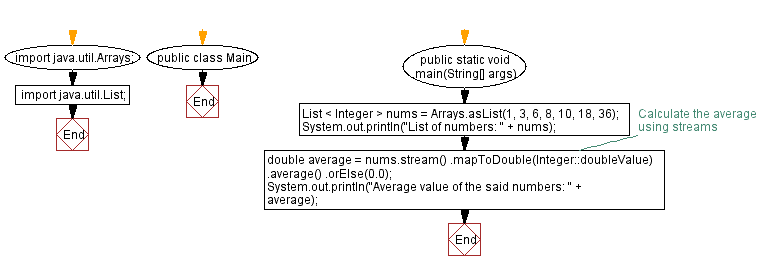﻿ Java Program: Calculate Average of Integers using Streams

# Java Program: Calculate Average of Integers using Streams

## Java Stream: Exercise-1 with Solution

Write a Java program to calculate the average of a list of integers using streams.

Sample Solution:

Java Code:

``````import java.util.Arrays;
import java.util.List;

public class Main {
public static void main(String[] args) {
List < Integer > nums = Arrays.asList(1, 3, 6, 8, 10, 18, 36);
System.out.println("List of numbers: " + nums);

// Calculate the average using streams
double average = nums.stream()
.mapToDouble(Integer::doubleValue)
.average()
.orElse(0.0);

System.out.println("Average value of the said numbers: " + average);
}
}
```
```

Sample Output:

```List of numbers: [1, 3, 6, 8, 10, 18, 36]
Average value of the said numbers: 11.714285714285714
```

Explanation:

In the above exercise, we have a list of integers (1, 3, 6, 8, 10, 18, 36). Using streams, we convert each integer to a double using the mapToDouble method and calculate the average through the average() method, and finally retrieve the average value using the orElse method in case the stream is empty. The average value is then printed to the console.

Flowchart:Java Code Editor:

Improve this sample solution and post your code through Disqus

What is the difficulty level of this exercise?

Test your Programming skills with w3resource's quiz.

﻿

## Java: Tips of the Day

Converts a string from camelcase:

```public static String fromCamelCase(String input, String separator) {
return input
.replaceAll("([a-z\\d])([A-Z])", "\$1" + separator + "\$2")
.toLowerCase();
}
```

Ref: https://bit.ly/3u09A9E

We are closing our Disqus commenting system for some maintenanace issues. You may write to us at reach[at]yahoo[dot]com or visit us at Facebook